# WO2017002441A1 - Route prediction device - Google Patents

## Info

Publication number
WO2017002441A1
WO2017002441A1 PCT/JP2016/063161 JP2016063161W WO2017002441A1 WO 2017002441 A1 WO2017002441 A1 WO 2017002441A1 JP 2016063161 W JP2016063161 W JP 2016063161W WO 2017002441 A1 WO2017002441 A1 WO 2017002441A1
Authority
WO
WIPO (PCT)
Prior art keywords
unit
route
predicted
likelihood
vehicle
Prior art date
Application number
PCT/JP2016/063161
Other languages
French (fr)
Japanese (ja)
Inventor

Original Assignee

Priority date (The priority date is an assumption and is not a legal conclusion. Google has not performed a legal analysis and makes no representation as to the accuracy of the date listed.)
Filing date
Publication date
Priority to PCT/JP2015/069131 priority Critical patent/WO2017002258A1/en
Priority to JPPCT/JP2015/069131 priority
Application filed by 三菱電機株式会社 filed Critical 三菱電機株式会社
Publication of WO2017002441A1 publication Critical patent/WO2017002441A1/en

• 238000004364 calculation methods Methods 0.000 claims abstract description 60
• 230000001133 acceleration Effects 0.000 claims description 13
• 230000000875 corresponding Effects 0.000 claims description 5
• 239000000203 mixtures Substances 0.000 description 19
• 238000000034 methods Methods 0.000 description 7
• 230000000694 effects Effects 0.000 description 3
• 230000001276 controlling effects Effects 0.000 description 1

## Images

•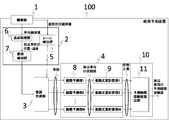•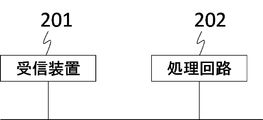•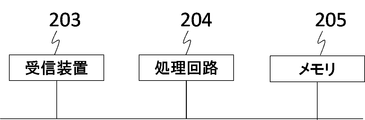•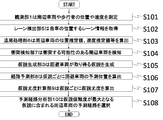•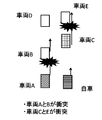•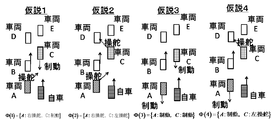•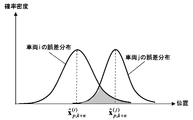•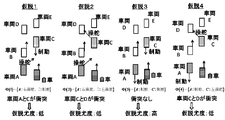••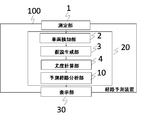•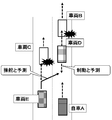•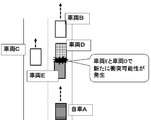•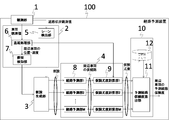## Classifications

• GPHYSICS
• G08SIGNALLING
• G08GTRAFFIC CONTROL SYSTEMS
• G08G1/00Traffic control systems for road vehicles
• G08G1/16Anti-collision systems
• G08G1/166Anti-collision systems for active traffic, e.g. moving vehicles, pedestrians, bikes
• GPHYSICS
• G08SIGNALLING
• G08GTRAFFIC CONTROL SYSTEMS
• G08G1/00Traffic control systems for road vehicles
• G08G1/123Traffic control systems for road vehicles indicating the position of vehicles, e.g. scheduled vehicles; Managing passenger vehicles circulating according to a fixed timetable, e.g. buses, trains, trams
• G08G1/133Traffic control systems for road vehicles indicating the position of vehicles, e.g. scheduled vehicles; Managing passenger vehicles circulating according to a fixed timetable, e.g. buses, trains, trams within the vehicle ; Indicators inside the vehicles or at stops
• GPHYSICS
• G08SIGNALLING
• G08GTRAFFIC CONTROL SYSTEMS
• G08G1/00Traffic control systems for road vehicles
• G08G1/16Anti-collision systems
• GPHYSICS
• G08SIGNALLING
• G08GTRAFFIC CONTROL SYSTEMS
• G08G1/00Traffic control systems for road vehicles
• G08G1/16Anti-collision systems
• G08G1/164Centralised systems, e.g. external to vehicles
• HELECTRICITY
• H04ELECTRIC COMMUNICATION TECHNIQUE
• H04WWIRELESS COMMUNICATION NETWORKS
• H04W4/00Services specially adapted for wireless communication networks; Facilities therefor
• H04W4/30Services specially adapted for particular environments, situations or purposes
• H04W4/40Services specially adapted for particular environments, situations or purposes for vehicles, e.g. vehicle-to-pedestrians [V2P]
• H04W4/44Services specially adapted for particular environments, situations or purposes for vehicles, e.g. vehicle-to-pedestrians [V2P] for communication between vehicles and infrastructures, e.g. vehicle-to-cloud [V2C] or vehicle-to-home [V2H]

## Abstract

This route prediction device is characterized by the provision of: a measurement unit that measures an area including a host vehicle and other moving vehicles; a vehicle detection unit that, on the basis of the measurement result measured by the measurement unit, detects at least two peripheral vehicles with which there is a possibility the host vehicle may collide; a hypothesis generation unit that generates a plurality of hypotheses for avoiding collision with the at least two peripheral vehicles detected by the vehicle detection unit; a likelihood calculation unit for calculating a likelihood indicating the probability that each of the plurality of hypotheses generated by the hypothesis generation unit will occur; and a predicted route analysis unit that analyzes the predicted routes of the at least two peripheral vehicles on the basis of the likelihood calculated by the likelihood calculation unit and outputs the analysis result. As a result of this configuration, it is possible to calculate the predicted routes of a plurality of vehicles without contradiction when there is a possibility of collision with a plurality of peripheral vehicles, and to improve the prediction performance with respect to the routes of peripheral vehicles.

## Description

Route prediction device

The present invention relates to a route predicting device for predicting a traveling route of a moving object.

In recent years, technology for predicting a safe route that avoids collisions between moving bodies is required in various fields such as vehicle driving support systems and air traffic control. For example, in a vehicle driving support system, the position of an obstacle such as a vehicle or a stop existing around the host vehicle is acquired by a sensor such as a millimeter wave radar, a laser radar, or a camera mounted on the host vehicle. Based on the relative distance and relative speed of the vehicle, a technology has been developed for controlling the host vehicle and preventing the collision after judging the collision risk. In addition, as a more advanced technology, automatic driving technology that recognizes the surrounding environment by the sensor, automatically operates the steering wheel and brakes without the driver's operation, and reaches the destination is being developed. .

Patent Document 1 discloses a route generation method that considers the interaction between a plurality of vehicles and a control amount evaluation method for the host vehicle. However, since an optimal predicted route is calculated based on a preset control rule, there is a problem that the predicted route is erroneous when the surrounding vehicle does not follow the control rule. In addition, since sensor errors when observing surrounding vehicle positions are not taken into account, there is a problem of erroneous prediction paths when there is an influence of sensor errors. Furthermore, there are many parameters to be set, such as giving the estimated speed for each surrounding vehicle as a parameter, and there is a concern that the design is complicated.

In Patent Document 2, a plurality of predicted routes of a vehicle are generated in advance, and a route that best meets a predetermined selection criterion is selected for the generated predicted route by calculating the degree of interference of the predicted route between the vehicle and the surrounding vehicles. A method is disclosed. However, when calculating the predicted route for all the surrounding vehicles, it is necessary to calculate the degree of interference in consideration of all the predicted routes of other surrounding vehicles with respect to the predicted route of one surrounding vehicle, and the calculation load is enormous. There is concern about becoming. Moreover, although the method for calculating only the predicted route of the host vehicle is disclosed, the method for calculating the predicted route of the surrounding vehicle is not disclosed or suggested.

The problem when calculating the predicted route in a situation where a plurality of surrounding vehicles are in close proximity will be described more specifically. Assume that the vehicles E and D collide with the vehicles C and B, which are the preceding vehicles, as shown in FIG. In addition, vehicle E has a high probability of steering and changing to the right lane to avoid a collision with vehicle C, and vehicle D has no space to change to the left lane, so there is a probability of braking and avoiding a collision. Suppose it is expensive. However, when a path for avoiding a collision with a preceding vehicle is predicted by the vehicle E being steered and the vehicle D being braked as shown in FIG. 12, the possibility of a collision between the vehicles D and E occurs. In this regard, even if a collision between the vehicles D and E is avoided by re-prediction, there is another possibility that another collision possibility occurs, and a predicted path for avoiding all possible collisions cannot be calculated.

Japanese Patent No. 3714258 Japanese Patent No. 4353192

The conventional route prediction apparatus predicts the route of one neighboring vehicle by calculating the degree of interference in consideration of all the predicted routes of other neighboring vehicles with respect to the predicted route of one neighboring vehicle. In addition, routes of a plurality of surrounding vehicles are predicted individually. However, in the conventional route prediction device, since the degree of interference with the combination of the predicted route of one neighboring vehicle and the predicted route of another neighboring vehicle is not calculated, route prediction is performed in consideration of the mutual movement of a plurality of neighboring vehicles. Absent. As a result, inconsistencies such as overlapping of route predictions of a plurality of surrounding vehicles may occur, and it is a problem that the routes of the plurality of surrounding vehicles cannot be accurately predicted. In addition, since the degree of interference is calculated in consideration of all predicted routes of other peripheral vehicles with respect to the predicted route of one peripheral vehicle, a large calculation load is also a problem.

The present invention has been made to solve the above-described problem, and in the case where a collision may occur in a plurality of vehicles in the future, it is possible to calculate the predicted routes of the plurality of vehicles without contradiction while reducing the calculation load. Objective.

The route prediction device according to the present invention is capable of colliding with the own vehicle based on an observation unit that observes the position of the own vehicle and the position and speed of the surrounding vehicle of the own vehicle, and an observation result observed by the observation unit. A vehicle detection unit for detecting at least two neighboring vehicles having a characteristic, a hypothesis generation unit for generating a plurality of hypotheses for avoiding a collision of at least two neighboring vehicles detected by the vehicle detection unit, and the hypothesis generation unit A likelihood calculating unit for calculating likelihood indicating the probability of occurrence of each of the plurality of hypotheses generated in step (b), and a predicted route of the at least two neighboring vehicles based on the likelihood calculated by the likelihood calculating unit. And a predicted path analysis unit that outputs the analysis result.

According to this invention, when a collision may occur in the plurality of surrounding vehicles in the future, it is possible to calculate the predicted routes of the plurality of vehicles without any contradiction while reducing the calculation load.

The figure which shows the structure of the route prediction apparatus 100 which concerns on Embodiment 1 of this invention. The figure which shows the hardware structural example of the path | route prediction apparatus 100 which concerns on Embodiment 1 of this invention. The figure which shows the other hardware structural example of the path | route prediction apparatus 100 which concerns on Embodiment 1 of this invention. The flowchart which shows the process in the route estimation apparatus 100 which concerns on Embodiment 1 of this invention. An example of the vehicle situation handled with the hypothesis generation part 3 in Embodiment 1 of this invention. The collision avoidance hypothesis handled by the hypothesis generation unit 3 according to Embodiment 1 of the present invention. The probability distribution of the vehicle in Embodiment 1 of this invention. The figure which shows the hypothesis likelihood with respect to each hypothesis in Embodiment 1 of this invention. The figure which shows the structure of the route estimation apparatus 100 which concerns on Embodiment 2 of this invention. The figure which shows the structure of the route prediction apparatus 100 which concerns on Embodiment 3 of this invention. An example of the road condition where multiple vehicles are mixed in the prior art. An example of mispredicting when multiple vehicles are avoided in the prior art. The figure which shows the structure of the route prediction apparatus 100 which concerns on Embodiment 4 of this invention.

Embodiment 1 FIG.
Embodiments of the present invention will be described below.

FIG. 1 is a configuration diagram illustrating a route prediction apparatus 100 according to the first embodiment. As illustrated in FIG. 1, the route prediction device 100 according to the present exemplary embodiment includes an observation unit 1, a vehicle detection unit 2, a hypothesis generation unit 3, a likelihood calculation unit 4, and a predicted route analysis unit 10. Here, the observation unit 1 uses a sensor such as a millimeter wave radar, a laser radar, an optical camera, an infrared camera, and a communication device that receives GPS positions of surrounding vehicles and pedestrians, and the like, Measure the position and speed of vehicles and pedestrians. The vehicle detection unit 2 has a function of detecting the own vehicle and at least two neighboring vehicles having a possibility of collision based on the observation result observed by the observation unit 1, and includes a lane detection unit 5, a tracking processing unit 6, a collision A detection unit 7 is provided. The hypothesis generation unit 3 has a function of generating a plurality of hypotheses for avoiding a collision between two surrounding vehicles detected by the vehicle detection unit 2. In addition, the likelihood calculation unit 4 has a function of calculating a likelihood indicating the probability of occurrence for each hypothesis generated by the hypothesis generation unit 3. The route prediction unit 8 and the hypothesis likelihood calculation unit 9 are I have. The predicted route analysis unit 10 has a function of analyzing a suitable predicted route based on the likelihood calculated by the likelihood calculating unit 4.

Observation unit 1, vehicle detection unit 2, hypothesis generation unit 3, likelihood calculation unit 4, lane detection unit 5, tracking processing unit 6, collision detection unit 7, route prediction unit in route prediction device 100 described in the first exemplary embodiment 8. Each function of the hypothesis likelihood calculation unit 9 and the predicted path analysis unit 10 is realized by a processing circuit (Processing Circuit). Even if the processing circuit is dedicated hardware, a CPU that executes a program stored in a memory (Central Processing Unit, central processing unit, processing unit, arithmetic unit, microprocessor, microcomputer, processor, DSP) It may be.

When the processing circuit is dedicated hardware, the path prediction device 100 includes a receiving device 201 including the observation unit 1 and a processing circuit 202, as shown in FIG. The processing circuit 202 may be, for example, a single circuit (a single circuit), a composite circuit (multi circuit), a programmed processor (a programmed processor), a parallel programmed processor (ASICCipSicCipSicCerciseCipSicCipSicCeCipSicCeCipSicCeCipSicCeCipSicCipSicCiP, and so on). ), FPGA (Field Programmable Gate Array), or a combination thereof. Observation unit 1, vehicle detection unit 2, hypothesis generation unit 3, likelihood calculation unit 4, lane detection unit 5, tracking processing unit 6, collision detection unit 7, route prediction unit 8, hypothesis likelihood calculation unit 9, prediction route analysis The functions of the respective units of the unit 10 may be realized by a processing circuit, or the functions of the respective units may be collectively realized by a processing circuit.

When the processing circuit is a CPU, the path prediction device 100 includes a receiving device 203, a processing circuit 204, and a memory 205 as shown in FIG. Observation unit 1, vehicle detection unit 2, hypothesis generation unit 3, likelihood calculation unit 4, lane detection unit 5, tracking processing unit 6, collision detection unit 7, route prediction unit 8, hypothesis likelihood calculation unit 9, prediction route analysis The function of the unit 10 is realized by software, firmware, or a combination of software and firmware. Software and firmware are described as programs and stored in a memory. The processing circuit 204 implements the functions of each unit by reading and executing a program stored in the memory. An example of these programs can be represented by steps S101 to S108 shown in FIG. These programs include an observation unit 1, a vehicle detection unit 2, a hypothesis generation unit 3, a likelihood calculation unit 4, a lane detection unit 5, a tracking processing unit 6, a collision detection unit 7, a route prediction unit 8, a hypothesis likelihood. It can also be said that the computer executes the procedures and methods of the calculation unit 9 and the predicted path analysis unit 10. Here, the memory 205 is, for example, a nonvolatile or volatile semiconductor memory such as RAM, ROM, flash memory, EPROM, EEPROM, a magnetic disk, a flexible disk, an optical disk, a compact disk, a mini disk, a DVD, or the like. Applicable.

Note that the observation unit 1, vehicle detection unit 2, hypothesis generation unit 3, likelihood calculation unit 4, lane detection unit 5, tracking processing unit 6, collision detection unit 7, route prediction unit 8, hypothesis likelihood calculation unit 9, prediction A part of the functions of the route analysis unit 10 may be realized by dedicated hardware, and part of the functions may be realized by software or firmware. For example, the function of the observation unit 1 is realized by a processing circuit as dedicated hardware, and the vehicle detection unit 2, hypothesis generation unit 3, likelihood calculation unit 4, lane detection unit 5, tracking processing unit 6, collision detection The functions of the unit 7, the route prediction unit 8, the hypothesis likelihood calculation unit 9, and the prediction route analysis unit 10 can be realized by the processing circuit reading and executing the program stored in the memory.

As described above, the processing circuit can realize the above functions by hardware, software, firmware, or a combination thereof.

FIG. 4 is a flowchart showing processing in the route prediction apparatus 100 according to Embodiment 1 of the present invention. The operation of the route prediction apparatus 100 will be described with reference to FIG. In FIG. 1, the observation unit 1 in the route prediction device 100 uses sensors such as millimeter wave radar, laser radar, optical camera, infrared camera, and a communication device that receives GPS positions of surrounding vehicles and pedestrians. The area including the own vehicle and other moving vehicles is observed, and the positions and speeds of surrounding vehicles and pedestrians are measured (S101). The lane detection unit 5 in the vehicle detection unit 2 recognizes a white line from a camera image or the like, and obtains lane information where the vehicle is located (S102). The tracking processing unit 6 in the vehicle detection unit 2 calculates a position estimation value of the surrounding vehicle, a speed estimation value, and an estimation error covariance matrix of the position and speed through tracking processing based on the position and speed acquired by the sensor. (S103).

The collision detection unit 7 in the vehicle detection unit 2 detects surrounding vehicles that may collide with each other (S104). For example, it may be detected based on the concept of TTC (Time To Collision). TTC is defined by equation (1). If TTC is equal to or less than a threshold, it is detected as a vehicle that may collide. Here, the vehicle i is traveling on the same lane as the vehicle j, and the vehicle i is a preceding vehicle of the surrounding vehicle j.

As another method, a predetermined area may be set around the own vehicle, and a vehicle having a predicted position after 1 to N steps in the area may be detected and regarded as a target vehicle. Here, N predicted positions up to N steps ahead are calculated as in equation (2). Here, Equations (2) to (5) are prediction equations based on a motion model (hereinafter, a constant velocity motion model) in which the vehicle travels with the current speed being constant. However, not only the constant velocity motion model but also a constant acceleration motion model may be predicted depending on the traveling state. *

The hypothesis generation unit 3 performs the following process when a plurality of collision possibilities are detected by the collision detection unit 7 and at least two surrounding vehicles having a collision possibility are detected. The hypothesis generation unit 3 first selects at least two neighboring vehicles (hereinafter referred to as avoiding vehicles) that have a collision possibility to take an avoidance action in order to avoid the plurality of collision possibilities. Next, a prediction scene (hereinafter referred to as a hypothesis) is generated from a combination of collision avoidance models that can be taken by at least two avoiding vehicles (S105). For example, as shown in FIG. 5, when the own vehicle and vehicles A to E exist and vehicles A and B and vehicles C and E collide, in order to avoid the collision between the following vehicles A and C, Avoid according to the collision avoidance model. Here, for example, a braking avoidance model, a left steering avoidance model, and a right steering avoidance model may be defined as the collision avoidance model. The braking avoidance model is a model that avoids collision by braking while maintaining the lane, and the left / right steering avoidance model is a model that changes lane to the left / right by inputting the steering amount and avoids collision. In the model, the braking amount or the steering amount is set so as not to exceed a predetermined limit value. In FIG. 5, since the traveling lane has two lanes, four hypotheses are generated from the combination of the collision avoidance models of the vehicles A and C as shown in FIG. In FIG. 6, since the traveling lane has two lanes, only the right steering model and the braking model are handled for the vehicle A, and the left steering model is not handled due to a contradiction with an actual route. Further, for the vehicle C, only the left steering model and the braking model are handled, and the right steering model is not handled due to a contradiction with an actual route. In this way, models that are inconsistent with the path that actually occurs are excluded, and only models that do not contradict are handled. In FIG. 6, all the combinations of models handled for the vehicles A and C are handled. However, when there is a contradiction with the actual route among the combinations of models handled for the vehicles A and C, The combination can also be eliminated. As a result, in the present embodiment, a combination of models that the vehicles A and C can take can be treated as a predicted scene (hypothesis). This hypothesis is stored in advance in a memory or the like, and a hypothesis suitable for the arrangement pattern of the avoiding vehicle can be read from the memory to extract the hypothesis.

Note that the conventional technology does not describe an example of generating a combination of avoidance models of at least two neighboring vehicles with a possibility of collision, and calculates the degree of interference with other neighboring vehicles individually for each neighboring vehicle. For this reason, it is necessary to calculate the degree of interference even in a state where contradictions occur, such as routes of a plurality of surrounding vehicles overlapping each other. On the other hand, in the present embodiment, by using a combination of avoidance models of at least two neighboring vehicles, it is possible to generate a hypothesis focused on only a combination of routes that at least two neighboring vehicles can take. As a result, it is possible to reduce the amount of calculation processing by advancing the calculation processing by focusing on a combination of necessary routes without causing a contradiction such as overlapping of routes that at least two neighboring vehicles can take.

The route prediction unit 8 in the likelihood calculation unit 4 calculates a predicted position up to N steps ahead based on the collision avoidance model for the avoidance vehicle for each hypothesis shown in FIG. 6 as a future predicted position (S106). For vehicles other than the avoidance vehicle, as described in the collision detection unit 7, the predicted position up to N steps ahead is calculated as a future predicted position on the assumption that the vehicle moves at a constant speed. However, there is no need to recalculate the predicted position that has already been calculated by the collision detection unit 7. As an example, a predicted position calculation method based on a collision avoidance model will be described. Based on the braking acceleration of the braking avoidance model, a temporary path (predicted position up to N steps ahead) is calculated as shown in Equation (6).

: Braking acceleration

The same calculation can be made for the left / right steering avoidance model. Here, since the predicted position of the vehicle with respect to steering differs depending on vehicle parameters such as the vehicle weight, the position of the center of gravity of the vehicle body, and the yaw moment of inertia, when the vehicle parameter is known, the predicted position is calculated in advance. In addition, when the vehicle parameter is unknown, a parameter estimated by a known learning algorithm or the like may be used.

The hypothesis likelihood calculation unit 9 in the likelihood calculation unit 4 calculates the hypothesis likelihood based on the temporary route calculated by the route prediction unit 8 for each hypothesis (S107). For example, regarding the calculation of hypothesis likelihood, likelihood evaluation is performed using the collision time. The number of predicted steps n min (i, j) that vehicles i and j are closest to is defined by equation (9), and the distance R min (i, j) between vehicles i and j is defined by equation (10).

When the distance R min (i, j) between the vehicles i and j is equal to or less than the threshold value, the vehicles i and j are considered to collide and the collision time is calculated.

When the distance R min (i, j) between the vehicles i and j exceeds the threshold, it is considered that there is no collision, and the collision time is set as a fixed value equal to or greater than the maximum prediction step number N.

Next, the likelihood evaluation value is defined by equation (13). The left side of Equation (13) is the likelihood of the mth hypothesis Φm, and Z (k) means the sensor observation value at the latest sample time k. Equation (13) means that the sum of collision times is taken for all combinations of surrounding vehicles. However, the above combination may be limited to only the two closest vehicles, or may be limited to only vehicles that are predicted to be on the same lane.

Moreover, regarding the calculation of the hypothesis likelihood, the likelihood evaluation may be performed in consideration of the sensor error or the error after the tracking process. First, the collision risk is calculated based on the position estimation error covariance matrix output from the tracking processing unit 6 and the position and speed estimation values. The value obtained by normalizing the difference between the predicted positions after n (n = 1,..., N) steps of the vehicles i and j at the sampling time k as shown in the equation (14), that is, the Mahalanobis square distance ε k + n is calculated.

The number of predicted steps n min (i, j) that minimizes the Mahalanobis square distance between vehicles i and j is given by Equation (17), and the Mahalanobis square distance minimum value ε min (i, j) between vehicles i and j is given by Equation (18). ).

Next, the likelihood evaluation value is defined by Expression (19). The right side of equation (19) means that the sum of Mahalanobis square distances is taken for all combinations of vehicles. However, it may be limited only to the two closest vehicles, or may be limited to only vehicles that are predicted to be on the same lane.

In calculating the hypothesis likelihood, the reciprocal of the degree of overlap may be used for the prediction error covariance matrix of the n steps ahead, using the overlap between prediction error distributions between vehicles as an index. For example, as shown in FIG. 7, the probability distribution of vehicles i and j is represented with the horizontal axis representing the position and the vertical axis representing the probability density function based on the error distribution. From FIG. 7, the degree of overlap corresponds to a range where the vehicle error distributions in FIG. 7 overlap (the hatched portion in FIG. 7). 7 is an area because the position is one-dimensional for convenience of explanation, but it is a volume on a two-dimensional plane.
Here, when the degree of overlap between the prediction error distributions between the vehicles i and j is D (i, j), the degree of overlap Dmax (i, j) in the number of prediction steps at which D (i, j) is maximum From the inverse, the likelihood evaluation value may be defined by equation (20).

Here, as the output of the hypothesis likelihood calculation unit 9, the hypothesis likelihood of each hypothesis is output. FIG. 8 is a diagram for explaining an output example by hypothesis likelihood calculation. From FIG. 8, Hypothesis 1 has collision with vehicles A and C, Hypothesis 2 has collision with vehicles C and D, Hypothesis 4 has low likelihood because vehicle C and D collide, and Hypothesis 3 has no collision. A result with a high hypothesis likelihood is obtained.

The predicted path analysis unit 10 first calculates the hypothesis reliability from the equation (21).

As a result, the reliability of the predicted path corresponding to each hypothesis can be grasped. The predicted route analysis unit 10 can output the analysis result related to the predicted route in various formats using the reliability of the predicted route. For example, the predicted route with the maximum reliability can be selectively output by selecting the predicted route of the surrounding vehicle included in the hypothesis Φ m having the maximum hypothesis reliability (S108). Moreover, it is also possible to output other formats from the predicted path analysis unit 10 using the reliability information. When the predicted route with the maximum reliability is output, the hypothetical reliability of hypothesis 3 is the highest in the example of FIG. 8, so that vehicle A selects the predicted route based on braking avoidance and vehicle C selects the predicted route based on braking avoidance. .

According to the first embodiment described above, the route prediction apparatus 100 is based on the observation unit 1 that observes the position and speed of the vehicle and its surrounding vehicles, and the observation results observed by the observation unit 1. A vehicle detection unit 2 that detects the host vehicle and at least two neighboring vehicles that may collide, and a hypothesis generation unit 3 that generates a plurality of hypotheses that avoid collision between at least two neighboring vehicles detected by the vehicle detection unit 2 And a likelihood calculation unit 4 that calculates the likelihood indicating the probability of occurrence of each of the plurality of hypotheses generated by the hypothesis generation unit 3 and at least 2 based on the likelihood calculated by the likelihood calculation unit 4 A route prediction apparatus comprising: a predicted route analysis unit that analyzes predicted routes of two neighboring vehicles and outputs the analysis result. With such a configuration, in the case where a plurality of surrounding vehicles may collide in the future, it is possible to cover the scenes that are assumed in reality without excess or deficiency by generating a prediction scene based on the vehicle motion. Since the predicted routes of a plurality of vehicles can be calculated without contradiction, the predicted performance of the routes of the surrounding vehicles is improved. In addition, it is not necessary to calculate an evaluation value such as an interference degree for an infinite number of routes as in the prior art, and it is possible to reduce a calculation load.

Further, according to the first embodiment, the vehicle detection unit 2 includes the lane detection unit 5 that detects the lane where the vehicle is located based on the observation result observed by the observation unit 1, and the observation that is observed by the observation unit 1. A tracking processing unit 6 that tracks the surrounding vehicle of the own vehicle based on the result, and a collision detection unit 7 that detects at least two surrounding vehicles that may collide from the surrounding vehicles that are tracked by the tracking processing unit 6; , Provided. With such a configuration, it is possible to detect at least two surrounding vehicles having a possibility of collision.

Further, according to the first embodiment, the likelihood calculating unit 4 predicts the future positions of at least two neighboring vehicles for each hypothesis generated by the hypothesis generating unit 3, and the route predicting unit 8 A hypothesis likelihood calculation unit 9 that calculates likelihoods indicating the likelihood of occurrence of each of a plurality of hypotheses generated by the hypothesis generation unit 3 based on the future positions of the at least two neighboring vehicles predicted in It is provided with. With such a configuration, it is possible to calculate the likelihood for the predicted route of at least two neighboring vehicles having a possibility of collision.

Further, according to the first embodiment, the predicted route analysis unit 10 selects and outputs the predicted routes of the at least two vehicles based on the likelihood calculated by the hypothesis likelihood calculation unit 9. . With such a configuration, a predicted route that is most likely to occur can be expressed as a prediction result of the route prediction device 100.

Embodiment 2. FIG.
In the first embodiment, the format for selectively outputting the predicted route with the maximum reliability from the predicted route analysis unit 10 is disclosed, but it is also possible to output the analysis result in other formats. In the second embodiment, a case where an analysis result different from that in the first embodiment is output will be described.

FIG. 9 is a configuration diagram showing the route prediction apparatus 100 according to the second embodiment. As shown in FIG. 9, the route prediction apparatus 100 of the present embodiment includes an observation unit 1, a lane detection unit 5, a tracking processing unit 6, a collision detection unit 7, a hypothesis generation unit 3, a route prediction unit 8, and a hypothesis likelihood calculation. 9, a predicted path analysis unit 10, and a predicted path reliability calculation unit 11 included in the predicted path analysis unit 10. Further, the hardware configuration for realizing the functions of the respective units in the route prediction apparatus 100 shown in FIG. 9 is represented by the configuration of FIG. 2 or FIG. 3 as in the first embodiment.

The predicted route reliability calculation unit 11 calculates an index representing the likelihood of occurrence of the predicted route of each vehicle as the predicted route reliability. For example, the reliability with which the vehicle i adopts the predicted route based on the collision avoidance model j is calculated by Expression (22). The left side of Equation (22) is the reliability with which the vehicle i adopts a predicted route based on the collision avoidance model j. Further, P (l i, j | Φ m ) in the equation (23) is a term relating to the combination, and is 1 when the predicted path is included in the hypothesis Φ m and 0 when not included.

Further, as shown in Expression (24), a value obtained by normalizing the predicted path based on the collision avoidance model j for the vehicle i with the reliability of the all collision avoidance model may be replaced with the predicted path reliability.

Since other processes are the same as those in the first embodiment, description thereof is omitted.

As described above, according to the second embodiment described above, the predicted route analysis unit 10 is based on the likelihood calculated by the likelihood calculating unit 4, and the predicted route reliability indicating the likelihood of the predicted routes of at least two neighboring vehicles. The predicted route reliability calculation unit 11 is provided for calculating the predicted route for each predicted route. With such a configuration, instead of determining a single predicted route for each vehicle as shown in the first embodiment, the user can intuitively output a quantitative value indicating the likelihood of occurrence of the predicted route. Understandable. There is an effect that it is easily used in other systems.

Embodiment 3 FIG.
The present embodiment shows a configuration in the case where the output from the route prediction apparatus 100 according to the first and second embodiments is displayed on the display unit.

FIG. 10 is a configuration diagram illustrating the route prediction apparatus 100 according to the third embodiment. As shown in FIG. 10, the route prediction apparatus 100 according to the third embodiment includes an observation unit 1, a signal processing unit 20, and a display unit 30, and the signal processing unit 20 is the vehicle shown in the first and second embodiments. A detection unit 2, a hypothesis generation unit 3, a likelihood calculation unit 4, and a predicted path analysis unit 10 are included. Further, the hardware configuration for realizing the function of each unit in the route prediction apparatus 100 shown in FIG. 10 is represented by the configuration of FIG. 2 or FIG. 3 as in the first embodiment.

In the route prediction apparatus 100 according to the present embodiment, the observation unit 1 observes an area including the own vehicle and other moving vehicles as in the first and second embodiments, and the observation results are used in the first and second embodiments. Similarly, the signal processing unit 20 generates a plurality of hypotheses indicating models in which at least two neighboring vehicles having a possibility of collision avoid the collision, and sets the predicted route reliability of the predicted route corresponding to each of the plurality of hypotheses. While calculating, outputting a predicted route reliability, the display unit 30 displays a predicted route that can be taken by at least two neighboring vehicles and a reliability corresponding to the predicted route on the display screen.

As described above, according to the third embodiment, the position of the own vehicle, the observation unit 1 that observes the position and speed of the surrounding vehicle of the own vehicle, and the observation result observed by the observation unit 1 are detected. A signal for generating a plurality of hypotheses indicating a collision avoidance model of at least two neighboring vehicles having a possibility of collision, and calculating a predicted route reliability of the predicted route of the at least two neighboring vehicles corresponding to each of the plurality of hypotheses A processing unit 20 and a display unit 30 for displaying the predicted route reliability and the predicted route reliability of the at least two neighboring vehicles based on the predicted route reliability calculated by the signal processing unit 20 are provided. . With such a configuration, the route prediction device 100 can visually grasp the predicted routes of the surrounding vehicles. Further, by displaying the predicted route by changing the color according to the predicted route reliability, the user can more accurately recognize the predicted route having the higher predicted route reliability. *

Embodiment 4 FIG.
In the first and second embodiments, there is no function for correcting the analysis result of the predicted route when irregular vehicle motion occurs, but the estimated speed value, acceleration estimated value, etc. of the surrounding vehicle estimated by the tracking process are used. Thus, it is possible to modify the hypothesis reliability and the prediction path reliability. In the first and second embodiments, the analysis result of the predicted path is output independently at each time, but it is also possible to analyze the predicted path in consideration of continuity in the time direction. In the fourth embodiment, a case where an analysis result different from those in the first and second embodiments is output will be described.

FIG. 13 is a configuration diagram illustrating the route prediction apparatus 100 according to the fourth embodiment. As illustrated in FIG. 13, the route prediction device 100 according to the present exemplary embodiment includes an observation unit 1, a lane detection unit 5, a tracking processing unit 6, a collision detection unit 7, a hypothesis generation unit 3, a route prediction unit 8, and a hypothesis likelihood calculation. 9, a predicted route analysis unit 10, a predicted route reliability calculation unit 11 and a reliability memory 12 provided in the predicted route analysis unit 10. Further, the hardware configuration for realizing the functions of the respective units in the route prediction apparatus 100 shown in FIG. 13 is represented by the configuration of FIG.

The hypothesis likelihood calculation unit 9 in the likelihood calculation unit 4 calculates the hypothesis likelihood based on the temporary route calculated by the route prediction unit 8 for each hypothesis. Here, the likelihood evaluation value P (Z (k) | Φ m ) related to the collision degree defined by the expression (19) or the expression (20) of the first embodiment and the likelihood evaluation value P (Trj Take the product of (k) | Φ m ) and calculate the hypothesis likelihood by the following equation.

Here, in the hypothesis Φ m (m = 1,..., M) at the sampling time k, the difference in time is clearly indicated by writing a subscript k in the upper right.

A method for calculating the likelihood evaluation value related to the avoidance trajectory of Equation (25) is described below. First, the likelihood calculation method for the right steering avoidance trajectory is shown below. When avoiding by right steering, speed is generated in the right direction with respect to the straight direction. Therefore, the likelihood of the right steering avoidance trajectory is calculated by Equation (26) using the lateral speed.

here,

The general lateral speed when changing lanes is set as a parameter.

Also, using the feature of moving to the right lane by avoiding the right, use equation (28) instead of equation (27) so that the likelihood increases as the lateral position is closer to the center line of the right adjacent lane. May be.

here,

The likelihood can be calculated for the left steering in the same way as the right avoidance.

Next, the avoidance trajectory likelihood calculation method related to braking avoidance will be described below. When avoiding by braking, acceleration occurs in the straight direction. Therefore, the likelihood of the braking avoidance trajectory is calculated by Equation (29) using the acceleration during braking.

here,

The general acceleration during braking is set as a parameter.

The vertical acceleration estimated value and the error standard deviation of the vertical acceleration estimated value can be calculated by a tracking process based on a uniform acceleration motion model. However, since it is difficult to calculate the likelihood when the estimation accuracy of the acceleration is not sufficient, a likelihood calculation method using speed is shown below.

here,

It is.

Like equation (32), the likelihood sum based on the collision avoidance model is calculated for all vehicles included in the hypothesis Φ m .

Here, I is a set of vehicle numbers, i is a vehicle number, and j is a collision avoidance model number. For example, in hypothesis 1 in FIG. 6, since vehicle A is right steering and vehicle C is braking, the likelihood of the right steering avoidance trajectory is calculated for vehicle A, and the likelihood of the braking avoidance trajectory is calculated for vehicle C. Take it.

The predicted path analysis unit 10 calculates the hypothesis reliability at the current sampling time k from Equation (33).

Here, the second term P (Φ m k | Φ m k-1 ) in the numerator of the equation (33) represents the transition probability of the hypothesis from the sampling time k-1 to the time k, unless there is any prior information. , Leave it as a parameter. Normally, you may set it to 1. The third term is the hypothesis reliability at the previous sampling time k−1, which is stored in the reliability memory 12 and read out for use.

The predicted route reliability calculation unit 11 calculates an index representing the likelihood of occurrence of the predicted route of each vehicle as the predicted route reliability. For example, the reliability with which the vehicle i adopts the predicted route based on the collision avoidance model j is calculated by Expression (34). The left side of the equation (34) is the reliability with which the vehicle i adopts the predicted route based on the collision avoidance model j.

Here, c is a constant, P (l i, j | Φ m k) is P (l i, j | Φ m) of the formula (23) is the same as.

Further, as in the first embodiment, the value obtained by normalizing the predicted route based on the collision avoidance model j for the vehicle i with the reliability of the all-collision avoidance model may be replaced as the predicted route reliability as shown in Expression (24). Be good. Since other processes are the same as those in the first and second embodiments, description thereof will be omitted.

As described above, according to the fourth embodiment described above, the likelihood calculating unit 4 calculates the hypothesis likelihood based on the estimated value output from the tracking processing unit 6 and the estimated error covariance matrix, and the predicted path analyzing unit 10 Based on the likelihood calculated by the likelihood calculating unit 4 and the hypothesis reliability at the previous sampling time, the predicted route reliability that calculates the predicted route reliability representing the likelihood of the predicted route of at least two neighboring vehicles is calculated for each predicted route. The degree calculation unit 11 is provided. With such a configuration, in addition to the effects of the first and second embodiments, the likelihood of occurrence of the predicted route of each vehicle is corrected according to the movement change of each vehicle, and the reliability with continuity in the time direction is further improved. By calculating, there is an effect that more accurate information can be provided to the user.

In the fourth embodiment, description of the same processing as in the first and second embodiments is omitted, but any combination of the first embodiment and the first and second embodiments is included in the present invention.

1: observation unit, 2: vehicle detection unit, 3: hypothesis generation unit, 4: likelihood calculation unit, 5: lane detection unit, 6: tracking processing unit, 7: collision detection unit, 8: route prediction unit, 9: Hypothesis likelihood calculation unit, 10: predicted route analysis unit, 11: predicted route reliability calculation unit, 12: reliability memory, 20: signal processing unit, 30: display unit, 100: route prediction device, 201, 203: reception Device, 202, 204: processing circuit, 205: memory

## Claims (10)

1. An observation unit for observing the position of the own vehicle and the position and speed of the surrounding vehicle of the own vehicle;
Based on the observation results observed by the observation unit, a vehicle detection unit that detects the host vehicle and at least two neighboring vehicles that may collide,
A hypothesis generation unit that generates a plurality of hypotheses in which at least two surrounding vehicles detected by the vehicle detection unit avoid a collision; and
A likelihood calculating unit that calculates a likelihood indicating the likelihood of occurrence of each of the plurality of hypotheses generated by the hypothesis generating unit;
Based on the likelihood calculated by the likelihood calculating unit, analyzing a predicted route of the at least two neighboring vehicles, and outputting a result of the analysis, a predicted route analyzing unit;
A route prediction apparatus comprising:
2. The vehicle detector is
A lane detection unit for detecting a lane where the vehicle is located based on an observation result observed by the observation unit;
A tracking processing unit that tracks the surrounding vehicle based on the observation result observed by the observation unit;
A collision detection unit that detects the at least two neighboring vehicles having a possibility of collision from the neighboring vehicles that are tracked by the tracking processing unit;
The route prediction apparatus according to claim 1, further comprising:
3. The likelihood calculating unit
A route prediction unit that predicts future positions of the at least two neighboring vehicles for each hypothesis generated by the hypothesis generation unit;
A hypothesis likelihood calculation that calculates a likelihood indicating a probability of occurrence of each of the plurality of hypotheses generated by the hypothesis generation unit based on future positions of the at least two neighboring vehicles predicted by the route prediction unit. And
The route prediction apparatus according to claim 1, further comprising:
4. The hypothesis likelihood calculator is
Based on the estimated speed and estimated speed error of the at least two neighboring vehicles predicted by the route predicting unit, the likelihood indicating the likelihood of occurrence of each of the plurality of hypotheses generated by the hypothesis generating unit The route prediction apparatus according to claim 3, wherein the route prediction apparatus calculates the route prediction apparatus.
5. The hypothesis likelihood calculator is
Based on the estimated acceleration and estimated acceleration error at the current time of the at least two neighboring vehicles predicted by the route predicting unit, the likelihood indicating the likelihood of occurrence of each of the plurality of hypotheses generated by the hypothesis generating unit is The route prediction apparatus according to claim 3, wherein the route prediction apparatus calculates the route prediction apparatus.
6. The said predicted route analysis part selects and outputs the predicted path | route of the said at least 2 surrounding vehicle based on the likelihood calculated by the said likelihood calculation part, The any one of Claim 1 thru | or 5 characterized by the above-mentioned. The route prediction apparatus described in 1.
7. The predicted route analysis unit calculates, for each predicted route, a predicted route reliability representing the likelihood of the predicted route of the at least two neighboring vehicles based on the likelihood calculated by the likelihood calculating unit. The route prediction apparatus according to claim 1, further comprising a calculation unit.
8. The predicted route analysis unit includes a predicted route reliability calculation unit that calculates a hypothesis reliability composed of predicted routes of the at least two neighboring vehicles based on the likelihood calculated by the likelihood calculation unit. The route prediction device according to claim 1, wherein the route prediction device is a feature of the route prediction device.
9. The predicted route reliability calculation unit calculates the predicted route reliability for each predicted route based on a hypothesis reliability composed of predicted routes of the at least two neighboring vehicles calculated at a previous time. The route prediction apparatus according to claim 7 or 8.
10. An observation unit for observing the position of the own vehicle and the position and speed of the surrounding vehicle of the own vehicle;
A plurality of hypotheses indicating a collision avoidance model of at least two of the surrounding vehicles with a possibility of collision detected based on the observation result observed by the observation unit are generated, and the at least two corresponding to each of the plurality of hypotheses A signal processing unit that calculates a predicted route reliability of the predicted route of the two neighboring vehicles, and a predicted route and a predicted route reliability of the at least two neighboring vehicles based on the predicted route reliability calculated by the signal processing unit. A display unit for displaying,
A route prediction apparatus comprising:
PCT/JP2016/063161 2015-07-02 2016-04-27 Route prediction device WO2017002441A1 (en)

## Priority Applications (2)

Application Number Priority Date Filing Date Title
PCT/JP2015/069131 WO2017002258A1 (en) 2015-07-02 2015-07-02 Route prediction device
JPPCT/JP2015/069131 2015-07-02

## Applications Claiming Priority (4)

Application Number Priority Date Filing Date Title
US15/739,650 US20180182245A1 (en) 2015-07-02 2016-04-27 Route prediction system
JP2017526206A JP6272566B2 (en) 2015-07-02 2016-04-27 Route prediction device
EP16817551.1A EP3319065A4 (en) 2015-07-02 2016-04-27 Route prediction device
CN201680037219.6A CN107710304A (en) 2015-07-02 2016-04-27 Path prediction meanss

## Publications (1)

Publication Number Publication Date
WO2017002441A1 true WO2017002441A1 (en) 2017-01-05

# Family

## Family Applications (2)

Application Number Title Priority Date Filing Date
PCT/JP2015/069131 WO2017002258A1 (en) 2015-07-02 2015-07-02 Route prediction device
PCT/JP2016/063161 WO2017002441A1 (en) 2015-07-02 2016-04-27 Route prediction device

## Family Applications Before (1)

Application Number Title Priority Date Filing Date
PCT/JP2015/069131 WO2017002258A1 (en) 2015-07-02 2015-07-02 Route prediction device

## Country Status (5)

US (1) US20180182245A1 (en)
EP (1) EP3319065A4 (en)
JP (1) JP6272566B2 (en)
CN (1) CN107710304A (en)
WO (2) WO2017002258A1 (en)

## Cited By (1)

* Cited by examiner, † Cited by third party
Publication number Priority date Publication date Assignee Title
WO2019072949A3 (en) * 2017-10-13 2019-05-23 Robert Bosch Gmbh Systems and methods for vehicle to improve an orientation estimation of a traffic participant

## Citations (2)

* Cited by examiner, † Cited by third party
Publication number Priority date Publication date Assignee Title
JP2010033352A (en) * 2008-07-29 2010-02-12 Nagoya Univ Lane change alarm and program
JP2014241036A (en) * 2013-06-11 2014-12-25 三菱電機株式会社 Vehicle driving assist system

## Family Cites Families (6)

* Cited by examiner, † Cited by third party
Publication number Priority date Publication date Assignee Title
JP4062353B1 (en) * 2006-11-10 2008-03-19 トヨタ自動車株式会社 Obstacle course prediction method, apparatus, and program
JP4623057B2 (en) * 2007-06-05 2011-02-02 トヨタ自動車株式会社 Moving area acquisition device for own vehicle
CN101943916B (en) * 2010-09-07 2012-09-26 陕西科技大学 Kalman filter prediction-based robot obstacle avoidance method
JP5189157B2 (en) * 2010-11-30 2013-04-24 株式会社豊田中央研究所 Moving object target state determination device and program
EP2851886B1 (en) * 2013-09-19 2018-04-11 Volvo Car Corporation Arrangement in a vehicle for providing vehicle driver support, a vehicle, and a method for providing vehicle driver support
CN104157167B (en) * 2014-08-28 2016-09-28 银江股份有限公司 A kind of vehicle collision avoidance method based on collaborative relative localization technology

## Patent Citations (2)

* Cited by examiner, † Cited by third party
Publication number Priority date Publication date Assignee Title
JP2010033352A (en) * 2008-07-29 2010-02-12 Nagoya Univ Lane change alarm and program
JP2014241036A (en) * 2013-06-11 2014-12-25 三菱電機株式会社 Vehicle driving assist system

## Non-Patent Citations (1)

* Cited by examiner, † Cited by third party
Title

## Cited By (1)

* Cited by examiner, † Cited by third party
Publication number Priority date Publication date Assignee Title
WO2019072949A3 (en) * 2017-10-13 2019-05-23 Robert Bosch Gmbh Systems and methods for vehicle to improve an orientation estimation of a traffic participant

## Also Published As

Publication number Publication date
CN107710304A (en) 2018-02-16
US20180182245A1 (en) 2018-06-28
EP3319065A4 (en) 2019-02-27
JPWO2017002441A1 (en) 2017-09-14
WO2017002258A1 (en) 2017-01-05
JP6272566B2 (en) 2018-01-31
EP3319065A1 (en) 2018-05-09

## Similar Documents

Publication Publication Date Title
US9274526B2 (en) Autonomous vehicle and method of estimating self position of autonomous vehicle
US9889858B2 (en) Confidence estimation for predictive driver assistance systems based on plausibility rules
CN103781685B (en) The autonomous drive-control system of vehicle
CN105799700B (en) Avoid collision control system and control method
JP5939357B2 (en) Moving track prediction apparatus and moving track prediction method
US9824586B2 (en) Moving object recognition systems, moving object recognition programs, and moving object recognition methods
US8610620B2 (en) Object detecting apparatus and object detecting method
EP2495713B1 (en) Driving support device
JP3427815B2 (en) Method and apparatus for selecting preceding vehicle, recording medium
DE102009043458B4 (en) Driving assistance system for vehicles
EP2302412B1 (en) System and method for evaluation of an automotive vehicle forward collision threat
US10246030B2 (en) Object detection apparatus and driving assistance apparatus
JP5765431B2 (en) Vehicle driving support system
EP2137041B1 (en) Method for the calculation of a collision-preventing trajectory for a driving maneuver of a vehicle
US9796378B2 (en) Vehicle travel path generating apparatus
JP4343536B2 (en) Car sensing device
JP4510554B2 (en) Three-dimensional object monitoring device
JP4980076B2 (en) Vehicle driving support device
JP4413957B2 (en) Moving object detection device and autonomous moving object
US20120330541A1 (en) Driving assistance device
JP5505427B2 (en) Collision position prediction device
JP6207723B2 (en) Collision prevention device
EP2169500B1 (en) Method of assessing vehicle paths in a road environment and a vehicle path assessment system.
JP6323565B2 (en) Driving assistance device
JP4906398B2 (en) In-vehicle road shape identification device, in-vehicle system, road shape identification method and periphery monitoring method

## Legal Events

Date Code Title Description
121 Ep: the epo has been informed by wipo that ep was designated in this application

Ref document number: 16817551

Country of ref document: EP

Kind code of ref document: A1

ENP Entry into the national phase in:

Ref document number: 2017526206

Country of ref document: JP

Kind code of ref document: A

WWE Wipo information: entry into national phase

Ref document number: 15739650

Country of ref document: US

NENP Non-entry into the national phase in:

Ref country code: DE

WWE Wipo information: entry into national phase

Ref document number: 2016817551

Country of ref document: EP